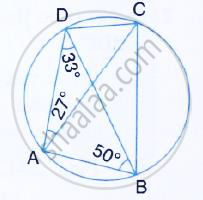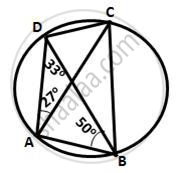Share

# Abcd is a Cyclic Quadrilateral in Which ∠Dac = 27° ; ∠Dba = 50° and ∠Adb = 33°. Calculate: ∠Dbc, - Mathematics

Course

#### Question

ABCD is a cyclic quadrilateral in which  ∠DAC = 27° ; ∠DBA = 50° and ∠ADB = 33°. Calculate : ∠DBC,#### Solution∠DBC =∠DAC = 27°
(Angle subtended by the same chord on the circle are equal)

Is there an error in this question or solution?